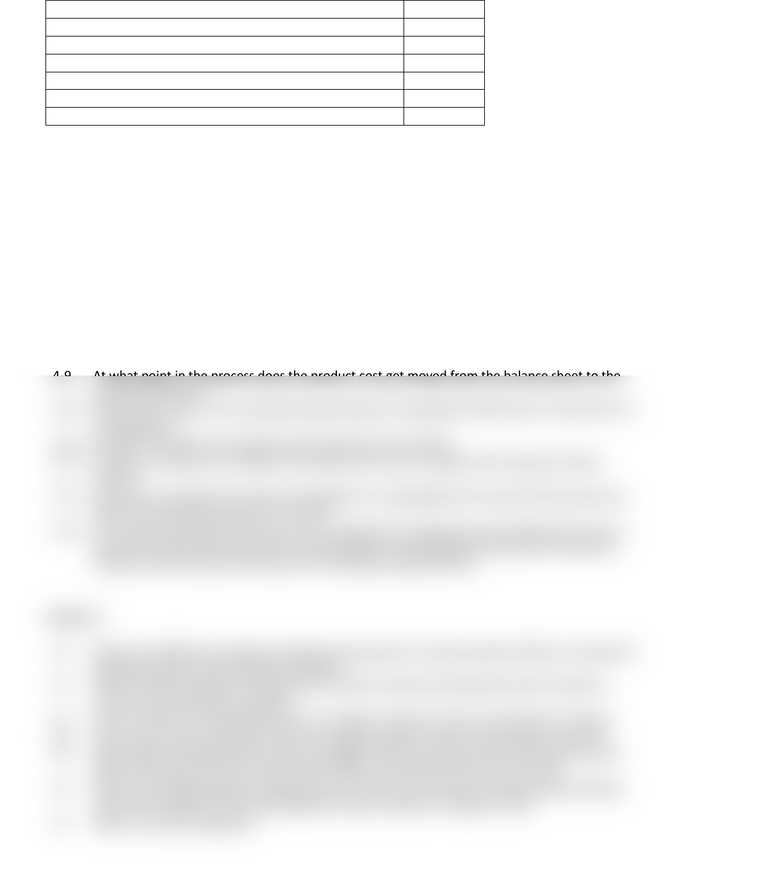# HIST 1402 Lecture Notes - Lecture 4: Total Absorption Costing, Income Statement, Fixed Cost

7 views2 pages
School
Department
CourseAcct 2102
Exam 2 Study Guide
Fall 2016
Exam Format
20 Multiple choice and true/false question x 3 points each
60 points
15 Matching questions x 1 point each
Traceable vs. common fixed costs
5 points
ABC cost levels
5 points
Identify relevant costs
5 points
Choose 3 of 4 Problems (details below) x 10 points each
30 points
Total
105 points
Chapter 4
4-1
What are some characteristics of job-order costing? What types of companies might use this
method?
4-2
The total cost of a job in a job-order costing system consists of what three costs?
4-3
When computing the cost of a job, is ACTUAL overhead or APPLIED overhead used?
4-4
What is a predetermined overhead rate?
4-5
What are the benefits of using an allocation base and a predetermined overhead rate?
4-6
What considerations should be made when choosing the allocation base (Hint: machines vs.
people)?
4-7
How is overhead applied to jobs? (i.e. what is the formula?)
4-8
Understand the three inventory accounts that are used in a job-order costing system and how
the costs flow through the accounts.
4-9
At what point in the process does the product cost get moved from the balance sheet to the
income statement?
4-10
What does it mean if the manufacturing overhead is overapplied? What does it mean when it's
underapplied?
4-11
Be able to calculate cost of goods sold using the direct method.
4-12
Be able to calculate cost of goods manufactured and cost of goods sold using the indirect
method.
4-13
How do you calculate the amount overapplied or underapplied for the period? How does this
affect cost of goods sold and net income?
4-14
You will have a problem where you must calculate the predetermined overhead rate and use
that rate to determine the amount of overapplied or underapplied overhead for the period.
Problem will be similar to #2 of your Ch 4 homework (Exercise 4-8).
Chapter 5
5-1
What is the difference between variable and absorption costing methods? What is included for
absorption that is not included for variable?
5-2
Which method (variable or absorption) is used for external reporting and which method is
used for internal decision making?
5-3
How is product cost calculated under the variable method? Under the absorption method?
5-4
How is net income calculated under the variable method? Under the absorption method?
5-5
Will variable or absorption net income be higher when we produce more units than we sell?
When we produce less units than we sell? When we produce the same as we sell?
5-6
When reconciling variable and absorption net income, do we add or subtract deferred (held)
costs from variable to arrive at absorption? Add or subtract "released" costs?
5-7
Unlock document

This preview shows half of the first page of the document.
Unlock all 2 pages and 3 million more documents.

## Document Summary

20 multiple choice and true/false question x 3 points each. Choose 3 of 4 problems (details below) x 10 points each. 4-6 what considerations should be made when choosing the allocation base (hint: machines vs. How is overhead applied to jobs? (i. e. what is the formula?) Understand the three inventory accounts that are used in a job-order costing system and how the costs flow through the accounts. 4-11 be able to calculate cost of goods sold using the direct method. 4-12 be able to calculate cost of goods manufactured and cost of goods sold using the indirect method. 4-14 you will have a problem where you must calculate the predetermined overhead rate and use that rate to determine the amount of overapplied or underapplied overhead for the period. Problem will be similar to #2 of your ch 4 homework (exercise 4-8). 5-15 you will have a problem where you have to prepare a segmented income statement.

## Get access

\$10 USD/m
Billed \$120 USD annuallyHomework Help
Study Guides
Textbook Solutions
Class Notes
Textbook Notes
Booster Class